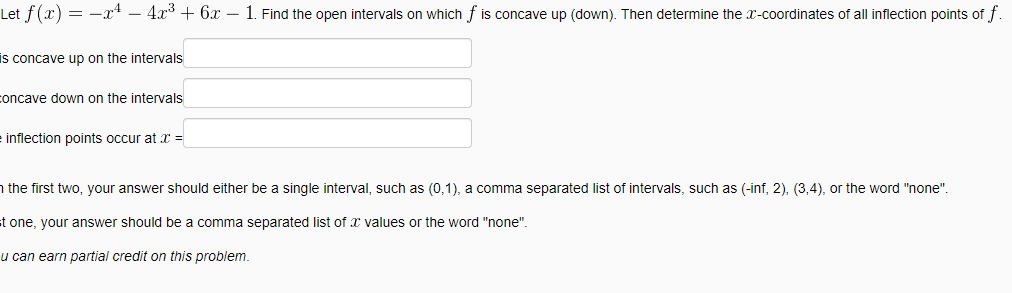# Let f (x) = -x – 4.x3 + 6x – 1. Find the open intervals on which f is concave up (down). Then determine the r-coordinates of all inflection points of f is concave up on the intervals toncave down on the intervals inflection points occur at x = h the first two, your answer should either be a single interval, such as (0,1), a comma separated list of intervals, such as (-inf, 2), (3,4), or the word "none". t one, your answer should be a comma separated list of x values or the word "none". u can earn partial credit on this problem.

Questionhelp_outlineImage TranscriptioncloseLet f (x) = -x – 4.x3 + 6x – 1. Find the open intervals on which f is concave up (down). Then determine the r-coordinates of all inflection points of f is concave up on the intervals toncave down on the intervals inflection points occur at x = h the first two, your answer should either be a single interval, such as (0,1), a comma separated list of intervals, such as (-inf, 2), (3,4), or the word "none". t one, your answer should be a comma separated list of x values or the word "none". u can earn partial credit on this problem. fullscreen

### Want to see this answer and more?

Experts are waiting 24/7 to provide step-by-step solutions in as fast as 30 minutes!*

*Response times may vary by subject and question complexity. Median response time is 34 minutes for paid subscribers and may be longer for promotional offers.
Tagged in
Math
Calculus

### Functions